Example Questions

2 Next →

Example Question #581 : Arithmetic

Choose the answer which best expresses the following percentage as a fraction, ensure that the answer is simplified: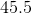%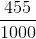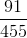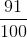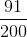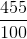Explanation:

To convert, simply first convert the percentage into a decimal: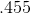Then, you can convert the decimal into a fraction, by putting the digits over one, followed by a number of zeroes equal to the number of digits:Both are divisible by five, so you can simplify:Example Question #582 : Arithmetic

Choose the answer which best converts the following percentage into a fraction: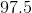%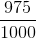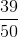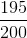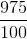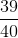Explanation:

To convert, first express the percentage as a decimal: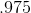Then, you can place the digits of the decimal over one followed by a number of zeroes equal to the number of digits:Both of these numbers are divisible by, so you can simplify:2 Next →

Tired of practice problems?

Try live online GRE prep today.